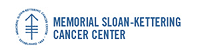Memorial Sloan-Kettering Cancer Center, Dept. of Epidemiology & Biostatistics Working Paper Series

Abstract

The concordance probability is used to evaluate the discriminatory power and the predictive accuracy of nonlinear statistical models. We derive an analytic expression for the concordance probability in the Cox proportional hazards model. The proposed estimator is a function of the regression parameters and the covariate distribution only and does not use the observed event and censoring times. For this reason it is asymptotically unbiased, unlike Harrell's c-index based on informative pairs. The asymptotic distribution of the concordance probability estimate is derived using U-statistic theory and the methodology is applied to a predictive model in lung cancer.

COinS